Courses

# Heat Transfer Class 11 Notes | EduRev

## Class 11 : Heat Transfer Class 11 Notes | EduRev

The document Heat Transfer Class 11 Notes | EduRev is a part of the Class 11 Course Physics Class 11.
All you need of Class 11 at this link: Class 11

HEAT TRANSFER

4. INTRODUCTION

Heat is energy in transit which flows due to temperature difference; from a body at higher temperature to a body at lower temperature. This transfer of heat from one body to the other takes place through three routes.

(i) Conduction
(ii) Convection
(iii) Radiation

(a) CONDUCTION

(i) Requires Medium

(ii) Energy is transmitted from one particle to another particle without displaced of particle

(iii) No transfer of particle

(b) CONVECTION

(i) Requires Medium

(ii) Energy is transfer through movement of the particle of medium.

(c) RADIATION

(i) Does not requires any medium

(ii) Energy is transfer through Electromagnetic waves.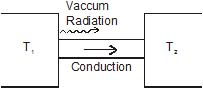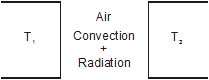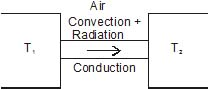5. CONDUCTION

Figure shows a rod whose ends are in thermal contact with a hot reservoir at temperature Ti and a cold reservoir at temperature T2. The sides of the rod are covered with insulating medium, so the transport of heat is along the rod, not through the sides. The molecules at the hot reservoir have greater vibrational energy. This energy is transferred by collisions to the atoms at the end face of the rod. These atoms in turn transfer energy to their neighbours further along the rod. Such transfer of heat through a substance in which heat is transported without direct mass transport is called conduction.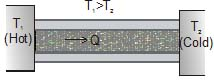Most metals use another, more effective mechanism to conduct heat. The free electrons, which move throughout the metal can rapidly carry energy from the hotter to cooler regions, so metals are generally good conductors of heat. The presence of 'free' electrons also causes most metals to be good electrical conductors. A metal rod at 5°C feels colder than a piece of wood at 5°C because heat can flow more easily from your hand into the metal.

Heat transfer occurs only between regions that are at different temperatures, and the rate of heat flow is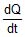. This rate is also called the heat current, denoted by H.

Experiments show that the heat current is proportional to the cross-section area A of the rod and to the temperature gradient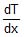, which is the rate of change of temperature with distance along the bar. In general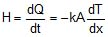The negative sign is used to makea positive quantity since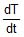is negative. The constant k, called the thermal conductivity is a measure of the ability of a material to conduct heat.

A substance with a large thermal conductivity k is a good heat conductor. The value of k depends on the temperature, increasing slightly with increasing temperature, but k can be taken to be practically constant throughout a substance if the temperature difference between its ends is not too great.

Let us apply Eq....(i) to a rod of length L and constant cross sectional area A in which a steady state has been reached. In a steady state the temperature at each point is constant in time. Hence.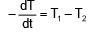Therefore, the heat DQ transferred in time Dt is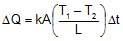Here, DT = temperature difference (TD) and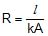= thermal resistance of the rod.

Important Points in conduction

1. Consider a section ab of a rod as shown in figure. Suppose Q1 heat enters into the section at 'a' and Q2 leaves at 'b', then Q2 < Q1 . Part of the energy Q1 - Q2 is utilized in raising the temperature of section ab and the remaining is lost to atmosphere through ab. If heat is continuously supplied from the left end of the rod, a stage comes when temperature of the section becomes constant. In that case, Q1 = Q2 if rod is insulated from the surroundings (or loss through ab is zero). This is called the steady state condition. Thus, in steady state temperature of different sections of the rod becomes constant (but not same).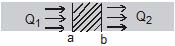Hence, in the figure :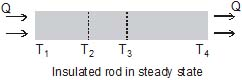T1 = constant, T2 = constant etc.

and T1 > T2 > T3 > T4

Now, a natural question arises, why the temperature of whole rod not becomes equal when heat is being continuously supplied ? The answer is : there must be a temperature difference in the rod for the heat flow, same as we require a potential difference across a resistance for the current flow through it.

In steady state, the temperature varies linearly with distance along the rod if it is insulated.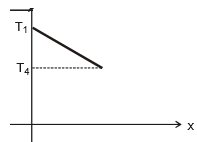2. Comparing equation number (iii), i.e., heat current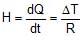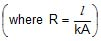with the equation, of current flow through a resistance,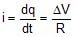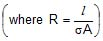We find the following similarities in heat flow through a rod and current flow through a resistance.

 Heat flow through a conducting rod Current flow through a resistance Heat current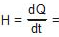rate of heat flow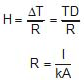Electric current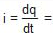rate of charge flow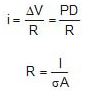k = thermal conductivity a = electrical conductivity

From the above table it is evident that flow of heat through rods in series and parallel is analogous to the flow of current through resistances in series and parallel. This analogy is of great importance in solving complicated problems of heat conduction.

Ex.7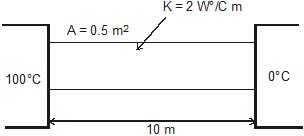Find out the heat current and temperature at any distance x.

Sol. R =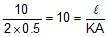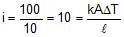and temperature at any distance x.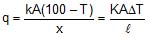⇒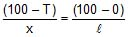100 l - T l = 100 x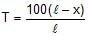RADIATION

6. RADIATION

The process of the transfer of heat from one place to another place without heating the intervening medium is called radiation. The term radiation used here is another word for electromagnetic waves. These waves are formed due to the superposition of electric and magnetic fields perpendicular to each other and carry energy.

Properties of Radiation :

(a) All objects emit radiations simply because their temperature is above absolute zero, and all objects absorb some of the radiation that falls on them from other objects.

(b) Maxwell on the basis of his electromagnetic theory proved that all radiations are electromagnetic waves and their sources are vibrations of charged particles in atoms and molecules.

(c) More radiations are emitted at higher temperature of a body and lesser at lower temperature.

(d) The wavelength corresponding to maximum emission of radiations shifts from longer wavelength to shorter wavelength as the temperature increases. Due to this the colour of a body appears to be changing. Radiations from a body at NTP has predominantly infrared waves.

(e) Thermal radiations travels with the speed of light and move in a straight line.

(f) Radiations are electromagnetic waves and can also travel through vacuum.

(g) Similar to light, thermal radiations can be reflected, refracted, diffracted and polarized.

(h) Radiation from a point source obeys inverse square law (intensity a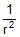)

6.1. PREVOST THEORY OF EXCHANGE

According to this theory, all bodies radiate thermal radiation at all temperatures. The amount of thermal radiation radiated per unit time depends on the nature of the emitting surface, its area and its temperature. The rate is faster at higher temperatures. Besides, a body also absorbs part of the thermal radiation emitted by the surrounding bodies when this radiation falls on it. If a body radiates more then what it absorbs, its temperature falls. If a body radiates less than what it absorbs, its temperature rises. And if the temperature of a body is equal to temperature of its surroundings it radiates at the same rate as it absorbs.

6.2 PERFECTLY BLACK BODY AND BLACK BODY RADIATION (FERY'S BLACK BODY)

A perfectly black body is one which absorbs all the heat radiations of whatever wavelength, incident on it. It neither reflects nor transmits any of the incident radiation and therefore appears black whatever be the colour of the incident radiation.

In actual practice, no natural object possesses strictly the properties of a perfectly black body. But the lamp-black and platinum black are good approximation of black body. They absorb about 99% of the incident radiation. The most simple and commonly used black body was designed by Fery. It consists of an enclosure with a small opening which is painted black from inside. The opening acts as a perfect black body. Any radiation that falls on the opening goes inside and has very little chance of escaping the enclosure before getting absorbed through multiple reflections. The cone opposite to the opening ensures that no radiation is reflected back directly.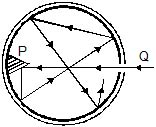6.3 ABSORPTION, REFLECTION AND EMISSION OF RADIATIONS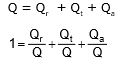where r = reflecting power, a = absorptive power and t = transmission power.
(i) r = 0, t = 0, a = 1, perfect black body
(ii) r = 1, t = 0, a = 0, perfect reflector
(iii) r = 0, t = 1, a = 0, perfect transmitter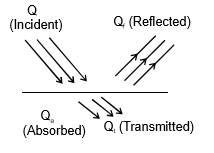(a) Absorptive power :

In particular absorptive power of a body can be defined as the fraction of incident radiation that is absorbed by the body.

a =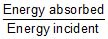As all the radiations incident on a black body are absorbed, a = 1 for a black body.

(b) Emissive power :

Consider a small area DA of a body emitting thermal radiation. Consider a small solid angle Dw about the normal to the radiating surface. Let the energy radiated by the area DA of the surface in the solid angle Dw in time Dt be DU. We define emissive power of the body as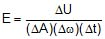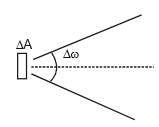Thus, emissive power denotes the energy radiated per unit area per unit time per unit solid angle along the normal to the area.

(c) Spectral Emissive power (El) :

Emissive power per unit wavelength range at wavelength l is known as spectral emissive power, El. If E is the total emissive power and El is spectral emissive power, they are related as follows,

E =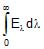and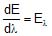(d) Emissivity :

e =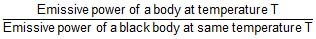=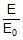7. STEFAN-BOLTZMANN'S LAW :

Consider a hot body at temperature T placed in an environment at a lower temperature T0. The body emits more radiation than it absorbs and cools down while the surroundings absorb radiation from the body and warm up. The body is losing energy by emitting radiations and this rate.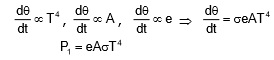and is receiving energy by absorbing radiations and this absorption rate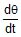=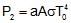Here, 'a' is a pure number between 0 and 1 indicating the relative ability of the surface to absorbs radiation from its surroundings. Note that this 'a' is different from the absorptive power 'a'. In thermal equilibrium, both the body and the surrounding have the same temperature (say Tc) and,

P1 = P2

or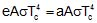or e = a

Thus, when T > T0, the net rate of heat transfer from the body to the surroundings is,

Net heat loss =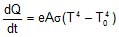or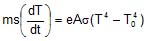⇒ Rate of cooling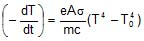or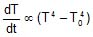Offer running on EduRev: Apply code STAYHOME200 to get INR 200 off on our premium plan EduRev Infinity!

## Physics Class 11

75 videos|295 docs|152 tests

,

,

,

,

,

,

,

,

,

,

,

,

,

,

,

,

,

,

,

,

,

;